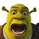צפיות ‎1451‎
MESA Phase (By John Ehlers ) is a cyclic indicator that predicts market short-term and mid-term movements. It would give you clear divergence signals or you can use it as a price reversal detection indicator.

Please share us any tricks or new useful methods that you determined by using this indicator.
הערות שחרור: "Horizontal Line" Bug fixed.
הערות שחרור:
• Source price modified.
• Offset added to get more accurate divergence points.
הערות שחרור:
• Bug fixes.

## תגובותDocop1
@Docop1, I will add the feature on update if others ask it too
השבgreywolf254
@greywolf254, I've tested it on many currencies with no problem. Which currency pair and TF do you use?
השב//@version=2
study("MESA Phase ")

DomCycle = input(15)
RealPart = 0
ImagPart = 0

for J = 0 to DomCycle - 1
Weight = close
if DomCycle != 0
RealPart := RealPart + cos(90 * J / DomCycle) * Weight * 2
ImagPart := ((ImagPart + sin(90 * J / DomCycle) * Weight) + (ImagPart + sin(180 * J / DomCycle) * Weight)) / 2

Phase = 0
if abs(RealPart) > .001
Phase := ((atan(ImagPart / RealPart)) - 0.685) * 100
else
Phase := ((90 * sign(ImagPart)) - 0.685) * 100

plot(0,color=gray)
plot(Phase,color=purple,linewidth=3)

upLine = input(5, minval=3, maxval=8, title="Upper Line Value?")
lowLine = input(-5, minval=-3, maxval=-8, title="Lower Line Value?")
p1 = plot(upLine, title= "Upper Line", style=solid, linewidth=1, color=red)
p2 = plot(lowLine, title= "Lower Line", style=solid, linewidth=1, color=green)

obLevel1 = input(title="Overbought", type=integer, defval=3)
osLevel1 = input(title="Oversold", type=integer, defval=-3)
obLevel2 = input(title="Overbought2", type=integer, defval=5)
osLevel2 = input(title="Oversold2", type=integer, defval=-5)
obLevel3 = input(title="Overbought3", type=integer, defval=8)
osLevel3 = input(title="Oversold3", type=integer, defval=-8)

hline(price=obLevel1, color = fuchsia)
hline(price=osLevel1, color = green)
hline(price=obLevel2, color = fuchsia)
hline(price=osLevel2, color = green)
hline(price=obLevel3, color = fuchsia)
hline(price=osLevel3, color = green)

Just a thought ;)
השבSchrekt
@Schrekt, Good work, tnx friend. Although the user should set upper line and lower line based on each currency.
השב